# 强化学习（六）：时序差分方法

时序差分（TD）方法结合了动态规划与蒙特卡洛的思想，其可以像蒙特卡洛方法一样直接从智能体与环境互动的经验中学习，而不需要知道环境的模型，其又可以像动态规划一样无须等待交互的结果，可以边交互边学习。总的来说，时序差分是基于已得到的其他状态的估计值来更新当前状态的价值函数。如果没有学习动态规划部分，可以浏览博文强化学习（四）：基于表格型动态规划算法的强化学习，如果没有学习蒙特卡洛方法，则可以浏览博文强化学习（五）：蒙特卡洛采样方法

我们知道，蒙特卡洛是基于采样的学习，从与环境的互动过程中所得到的经验作为求解贝尔曼方程的方法，然而，蒙特卡洛以及较强的分幕式设定在某些场景下并不适合，例如采样序列无限长，或者幕与幕之间没有明确的分界等情况。时序差分则结合动态规划的自举思想和蒙特卡洛的采样思想，使得其边采样边自举，不仅加快了学习的速度，也能适应诸多场景。本文将主要介绍TD（0）算法，即在采样期间，只将相邻的下一时刻状态估计值更新当前的价值函数，也就是说借用的估计值所处的时刻与目标更新的时刻差为1，亦称单步TD。在后续章节也会介绍TD（n），即多步TD。

#### 一、TD误差

我们已经非常熟悉强化学习的价值更新公式以及GPI思想，例如动态规划的策略预测：

v k + 1 ( s ) = ∑ a π ( a ∣ s ) ∑ s ′ , r p ( s ′ , r ∣ s , a ) [ r + γ v k ( s ′ ) ] v_{k+1}(s)=\sum_{a}\pi(a|s)\sum_{s',r}p(s',r|s,a)[r+\gamma v_{k}(s')]

Q ( S t , A t ) = Q ( S t , A t ) + W C ( S t , A t ) [ G t − Q ( S t , A t ) ] Q(S_t,A_t) = Q(S_t,A_t) + \frac{W}{C(S_t,A_t)}[G_t-Q(S_t,A_t)]

C ( S t , A t ) = C ( S t , A t ) + W C(S_t,A_t) = C(S_t,A_t) + W

（1）策略预测更新公式：

V ( S t ) = V ( S t ) + α [ G t − V ( S t ) ] V(S_t) = V(S_t) + \alpha[G_t - V(S_t)]

（2）策略控制更新公式：

Q ( S t , A t ) = Q ( S t , A t ) + α [ G t − Q ( S t , A t ) ] Q(S_t,A_t) = Q(S_t,A_t) + \alpha[G_t - Q(S_t,A_t)]

#### 二、时序差分TD（0）预测

上述已经介绍了时序差分的内容，根据GPI思想，我们先引入TD（0）预测算法。

V ( S t ) = V ( S t ) + α [ R t + 1 + γ V ( S t + 1 ) − V ( S t ) ] V(S_t) = V(S_t) + \alpha[R_{t+1} + \gamma V(S_{t+1}) - V(S_t)]

R + γ V ( S t + 1 ) R + \gamma V(S_{t+1}) 这一项就是所谓的将下一时刻的状态价值带有折扣的与当前时刻收益的和作为估计的实际价值，对比蒙特卡洛，这一部分则是需要累加所有的收益，而TD（0）只需要自举的将相邻的下一步作为估计，融合动态规划思想。TD（0）预测的算法流程如下：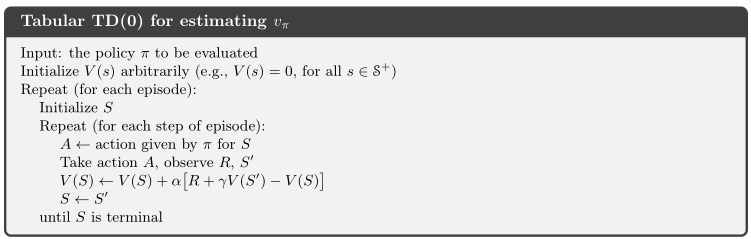v π ( s ) = E π [ G t ∣ S t = s ] v_{\pi}(s) = \mathbb{E}_{\pi}[G_t|S_t=s]

= E π [ R t + 1 + γ G t + 1 ∣ S t = s ] =\mathbb{E}_{\pi}[R_{t+1} + \gamma G_{t+1}|S_t=s]

= E π [ R t + 1 + γ v π ( S t + 1 ) ∣ S t = s ] =\mathbb{E}_{\pi}[R_{t+1} + \gamma v_{\pi}(S_{t+1})|S_t=s]

我们通过Sutton书上的一个示例来理解：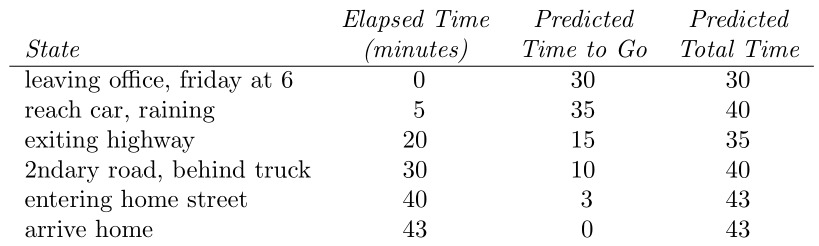在这个例子中，离开办公室，到达车库，离开高速路，行驶在普通公路，到达家门口和到家等都是状态，其实我们发现，驾驶员并不是到了家（最终状态）之后才对之前的每一个状态进行预估，在真实情况下也的确不现实，而驾驶员是在每一个状态之后，根据当前状态的情况，做出的临时预测，这一个过程就是时序差分的思想#### 三、时序差分策略控制

在GPI的策略控制阶段，我们依然分别通过同轨和离轨来讨论。

##### 3.1 时序差分同轨策略控制——SARSA

在同轨策略控制过程中，行动策略与目标策略是一致的，更新公式如下：

Q ( S t , A t ) = Q ( S t , A t ) + α [ R t + 1 + γ Q ( S t + 1 , A t + 1 ) − Q ( S t , A t ) ] Q(S_t,A_t) = Q(S_t,A_t) + \alpha[R_{t+1} + \gamma Q(S_{t+1},A_{t+1}) - Q(S_t,A_t)]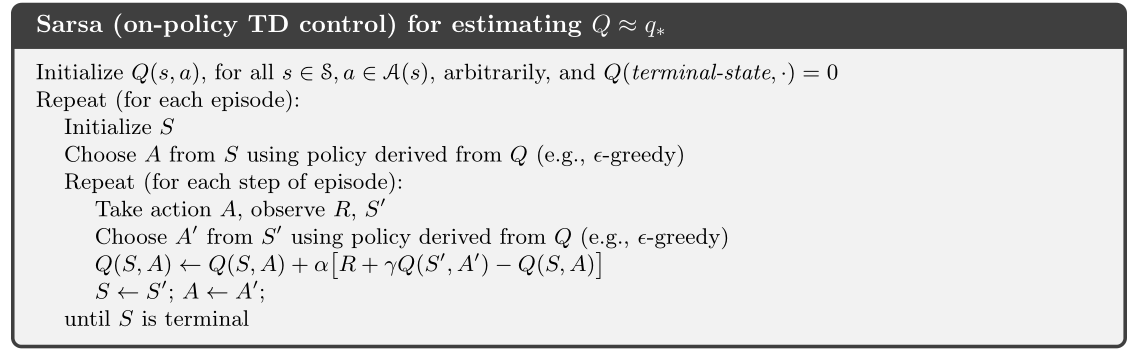##### 3.2 时序差分离轨策略控制——Q-Learning

Q学习是另一种TD（0）控制，也被称为离轨策略下的策略控制，其更新公式如下：

Q ( S t , A t ) = Q ( S t , A t ) + α [ R t + 1 + γ max ⁡ a Q ( S t + 1 , a ) − Q ( S t , A t ) ] Q(S_t,A_t) = Q(S_t,A_t) + \alpha[R_{t+1} + \gamma \max_{a}Q(S_{t+1},a) - Q(S_t,A_t)]##### 3.3 期望SARSA

另外再介绍一个策略控制方法——期望SARSA，其所描述的则是下一时刻的所有（状态-动作）二元组的期望值作为估计值，其回溯图如图：Q ( S t , A t ) = Q ( S t , A t ) + α [ R t + 1 + γ ∑ a π ( a ∣ S t + 1 ) Q ( S t + 1 , a ) − Q ( S t , A t ) ] Q(S_t,A_t) = Q(S_t,A_t) + \alpha[R_{t+1} + \gamma \sum_{a}\pi(a|S_{t+1})Q(S_{t+1},a) - Q(S_t,A_t)]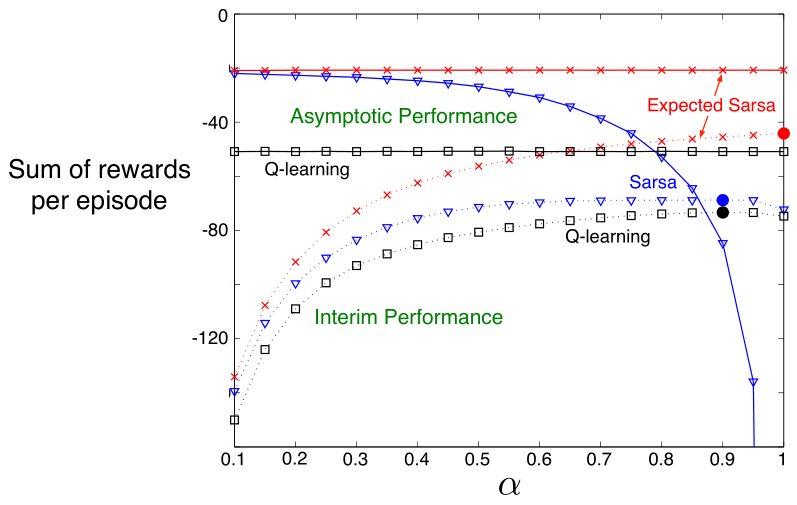最后总结一下三个控制策略的一些区别，SARSA是将遵循同一个策略，下一时刻的状态动作二元组的动作价值函数作为当前的估计值；Q学习则是在行动策略下的采样，用最大贪心策略对应的最大动作价值函数作为当前的估计值；期望SARSA则是将下一时刻所有的状态动作二元组的动作价值的期望值作为当前的估计值。从性能的排序来看，从长远性能来看，期望SARSA > Q > SARSA，从短期性能角度看，期望SARSA > SARSA > Q。因此说期望SARSA更优。

#### 四、最大化偏差与双学习

我们知道蒙特卡洛是无偏估计，而时序差分是一个有偏估计。因为时序差分是建立在对价值函数估计的基础上来预测，换句话说，本身执行采样的过程就是一种预测过程，而实际价值使用估计值则又是一个近似的过程，所以说时序差分是包含两次估计的方法，会产生一定的正偏差，也叫做最大化偏差。
解决这样的办法是采用双学习，我们以双Q学习为例，其算法流程如下所示：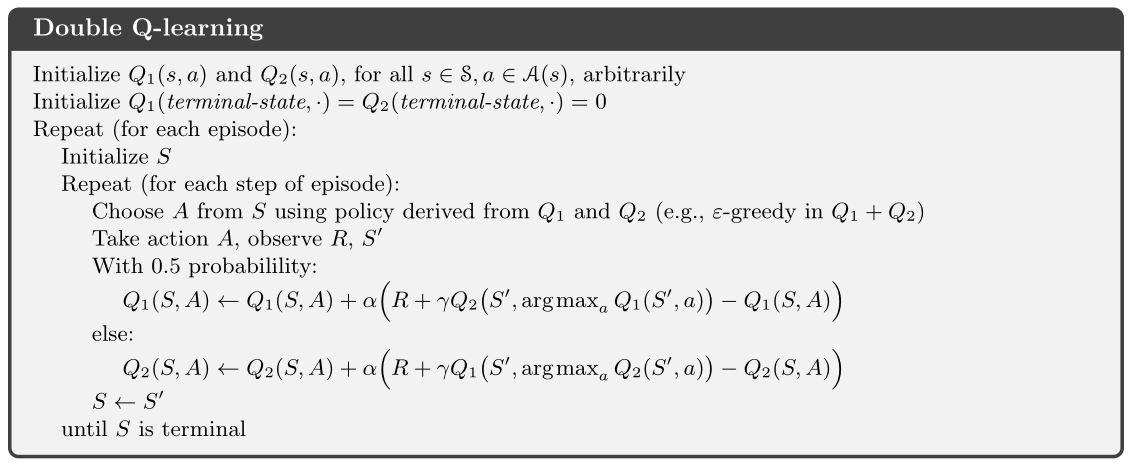#### 五、总结

时序差分法是结合了动态规划和蒙特卡洛两者思想，使用蒙特卡洛的采样方法，在经验中学习。但在每一步执行过程中，并不是等待完整的序列，而是边采样边学习。本文描述的是TD（0）单步法，其自举的范围只有一步，其实只自举一步未免显得智能体的眼光很短钱，事实上，蒙特卡洛也是一种特殊的时序差分，只不过其自举的是整个序列，虽然眼光最长远，但很耗时间。那有没有介于两个极端之间的呢？有！它就是TD（n），叫做n步自举法，也叫做多步引导法，其描述的则是多个步之后的价值作为当前时刻的估计值，简单的理解则是估计的范围不长也不短，眼光恰当正好，在一般情况下，我们也比较推荐使用TD（0）和TD（n）。

01-201万+
04-1344207-31117
07-287286
04-0885
03-16577
03-12112
08-2254
01-0849